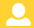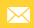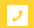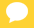# Method Of Measuring WSBC Ball Bearing Load

Time：2021.07.07  Source：Roller Bearings Suppliers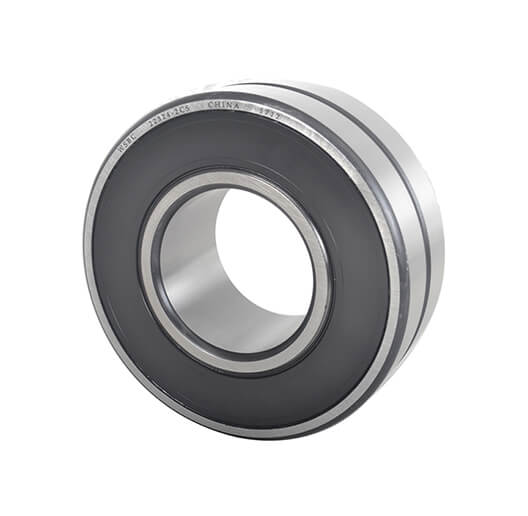1. The principle of the indirect measurement method of axial load

In the process of aero-engine design, according to the calculation method of the axial force of the engine rotor, the axial force of the rotor can be divided into two parts: the axial force of the disc cavity and the axial force of the runner. , The axial force of the disc cavity is the sum of the axial loads acting on the rotor system of each chamber in the engine air system, and the axial force of the runner is the sum of the axial loads on the turbine runner of the engine compressor. The calculation of each axial force component is mainly based on the momentum theorem.

The input parameters for calculating the axial force of the rotor mainly include: static pressure at the inlet of the runner, axial velocity, area, static pressure at the outlet of the runner, axial velocity, area, mass flow through the runner, cavity pressure, runner and disk The geometric parameters of the cavity, etc.

The indirect measurement method of the axial load of the ball bearing is based on the calculation method of the axial force of the rotor. The basic idea is to measure and superimpose the axial force of the disc cavity and the axial force of the runner for each component.

Wuxi Spark Bearing Co.,Ltd provides high quality WSBC BS2-2219-2CSK.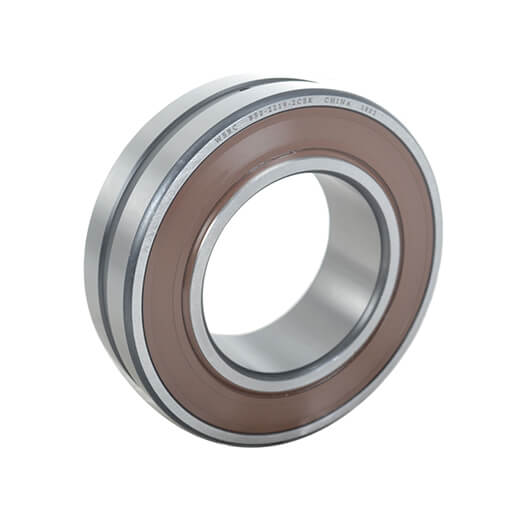For the axial force of the disc cavity, it can be calculated by real-time measurement of the cavity pressure of each cavity related to the engine rotor system, combined with the geometric dimensions of the corresponding disc cavity. However, due to the limitations and feasibility of the test system during the engine test run, it is impossible to completely measure the pressure of the air system chamber required by the engine, so relevant calculations must be performed. For example, the pressure of the disk cavity can be estimated through the flow pressure, back pressure and sealing form of the disk cavity, combined with certain empirical formulas and correction methods, and then after relevant calculations and processing, all the required air can be obtained. The static pressure of the disc cavity of the system realizes the real-time measurement and calculation of the axial force of the disc cavity. The key to the calculation lies in the selection of empirical formulas and correction parameters. The selection of these formulas and parameters directly affects the accuracy of the calculation of the axial force of the disc cavity. When dealing with the axial force of the disc cavity, the influence of the momentum generated by the air flow in the disc cavity on the axial force of the rotor is generally ignored.

For the runner axial force, real-time measurement of the runner axial force can be achieved by real-time measurement of the inlet and outlet static pressure and axial velocity of the relevant section of the compressor and turbine runner, combined with the calculation method of the runner axial force. The same as the test of the axial force of the disc cavity, usually the parameters of certain sections cannot be directly tested. For example, for some reasons, it is difficult to directly measure the turbine inlet parameters, but they can be calculated by inverse calculation of the turbine outlet parameters according to the working characteristics of the turbine. After knowing the compressor, turbine runner inlet and outlet static pressure and axial velocity, etc., according to the common working line of the engine, component characteristic line, etc., the inlet and outlet static pressure and axial velocity of each stage of the rotor blades are interpolated and fitted. To speed and so on. When the engine is working in different working conditions, the interpolation formula is quite different, so it is necessary to judge and calculate multiple sets of interpolation methods. Then calculate the axial force of each stage of the rotor blades according to a specific algorithm, and finally superimpose, and calculate the flow channel axial force of the entire rotor system in real time. The key to the calculation lies in the selection and correction of interpolation and fitting formulas. The axial force of the rotor of the engine is obtained by the superposition of the axial force of the disc cavity and the axial force of the runner.

In the indirect measurement of the axial force of the engine rotor, in order to display it in real time, it is necessary to prepare a special program for measurement based on the parameters in the engine measurement channel. When adopting the indirect measurement method of axial load, it is necessary to have many detailed parameters such as the performance and structure of each engine component, combined with the feasibility of the test system, for those parameters that are not easy to directly measure, it is necessary to flexibly use the measured parameters, engine Derive structural performance data. For the proposed axial load measurement algorithm, it is also necessary to adopt a variety of methods to check and modify it, and finally form an indirect measurement method for axial load suitable for a specific engine.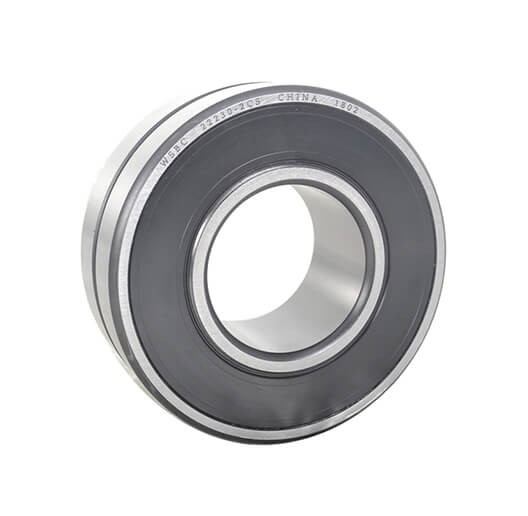2. Features of the indirect measurement method of axial load

(1) The measurement accuracy is controllable. If higher measurement accuracy is required, more comprehensive measurement parameters need to be proposed for measurement.

(2) Each component of the axial load can be measured. The axial load of the ball bearing is composed of several components superimposed, and the indirect measurement method of axial load can output each axial force component, which is beneficial to the analysis and adjustment of the axial force of the engine.

(3) The axial force of the rotor of the engine can be measured in real time. Through the indirect measurement program of the axial load, the axial force of the engine can be displayed in real time during the test run of the engine bench, which provides a basis for monitoring the condition of the engine.

(4) The measurement method is simple, no need to modify the engine structure. During the test run of the engine bench, many parameters need to be measured to verify the design level of the engine. This method selects relevant measurement parameters (some parameters can be appropriately supplemented) to measure the axial load of the ball bearing.

However, this method also has shortcomings. The most important thing is that a more comprehensive parameter measurement of the engine is required. In the engine trial production stage, a more comprehensive parameter measurement can be implemented. In the batch production stage, a large number of parameter measurement requirements cannot be met, and other methods are needed to achieve the measurement of the axial load of the ball bearing.

## Hot Topics

TEL：+86 0510-83220817

FAX：+86 0510-83212137

Mail：sales@spark-bearing.com